Скачать презентацию Introduction to Programming Using C Basic Computations

0d8e931d22c94e523ce8515d7713f84d.ppt

• Количество слайдов: 36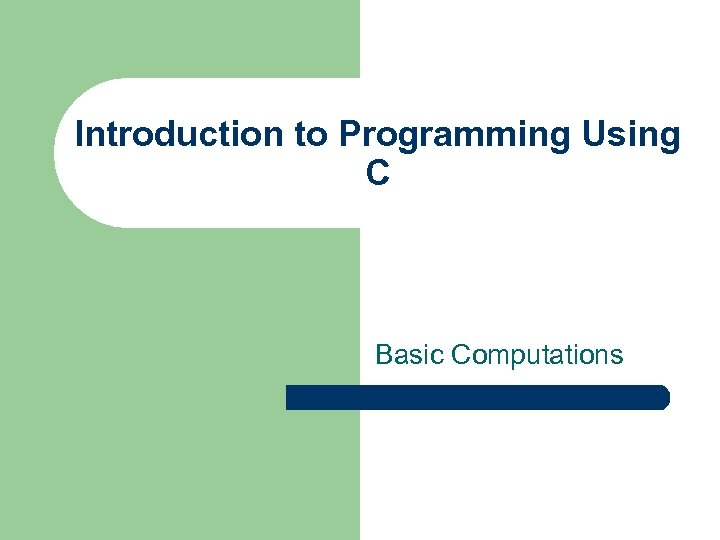Introduction to Programming Using C Basic Computations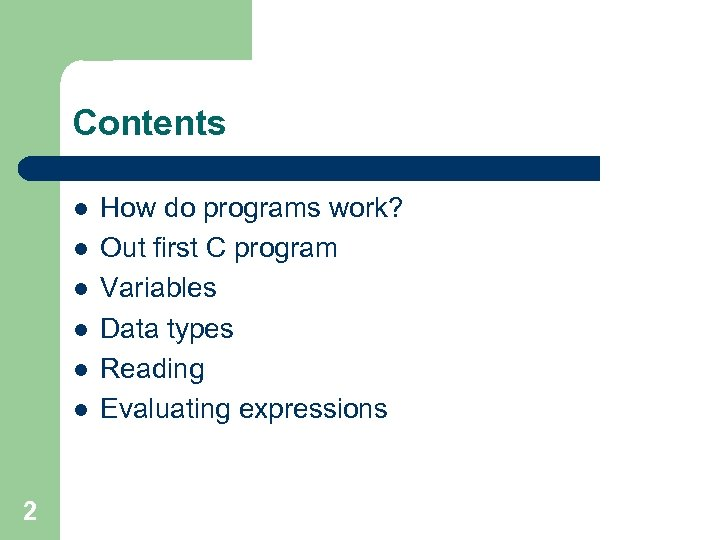Contents l l l 2 How do programs work? Out first C program Variables Data types Reading Evaluating expressions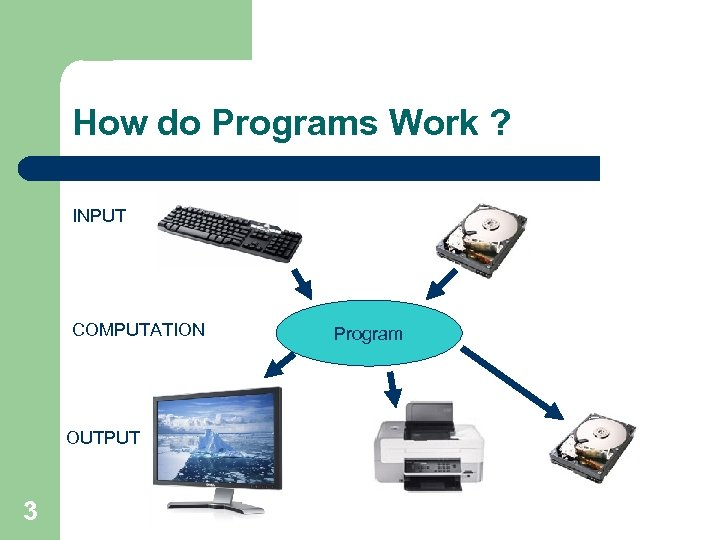How do Programs Work ? INPUT COMPUTATION OUTPUT 3 Program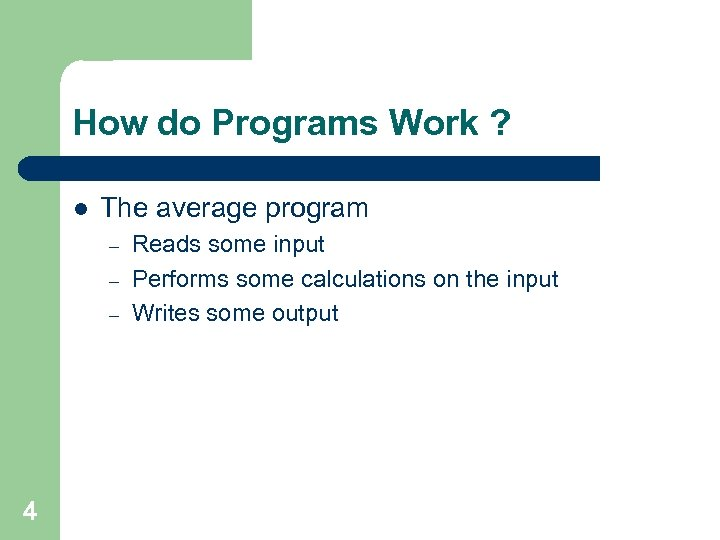How do Programs Work ? l The average program – – – 4 Reads some input Performs some calculations on the input Writes some output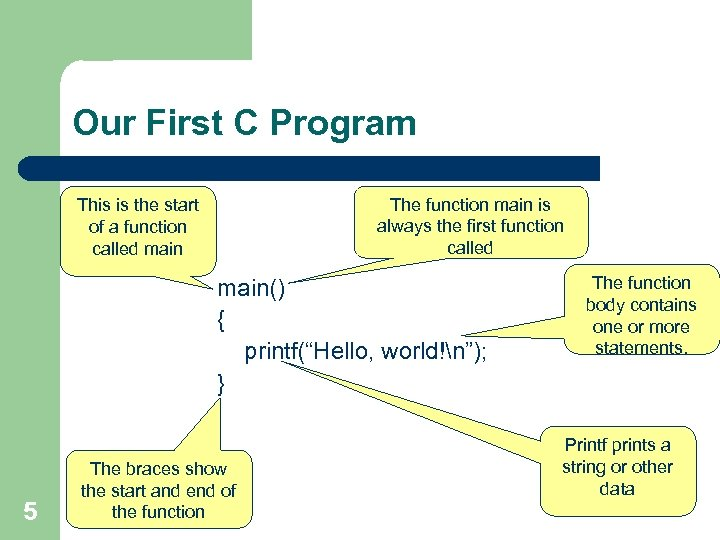Our First C Program The function main is always the first function called This is the start of a function called main() { printf(“Hello, world!n”); } 5 The braces show the start and end of the function The function body contains one or more statements. Printf prints a string or other data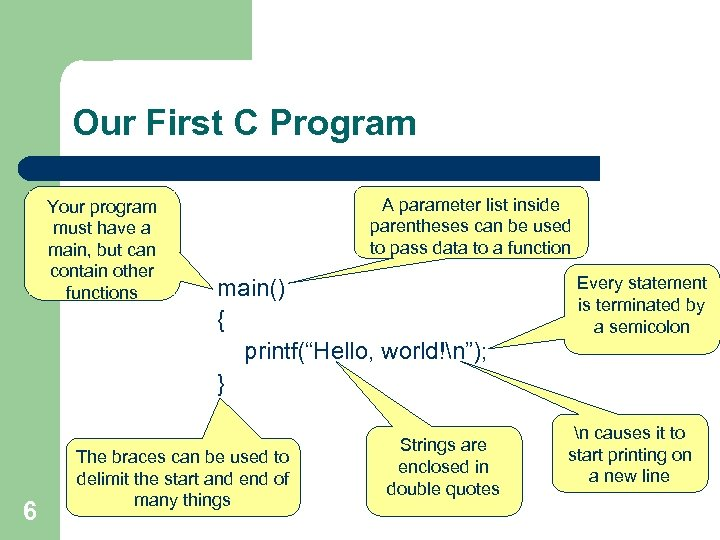Our First C Program Your program must have a main, but can contain other functions 6 A parameter list inside parentheses can be used to pass data to a function main() { printf(“Hello, world!n”); } The braces can be used to delimit the start and end of many things Strings are enclosed in double quotes Every statement is terminated by a semicolon n causes it to start printing on a new line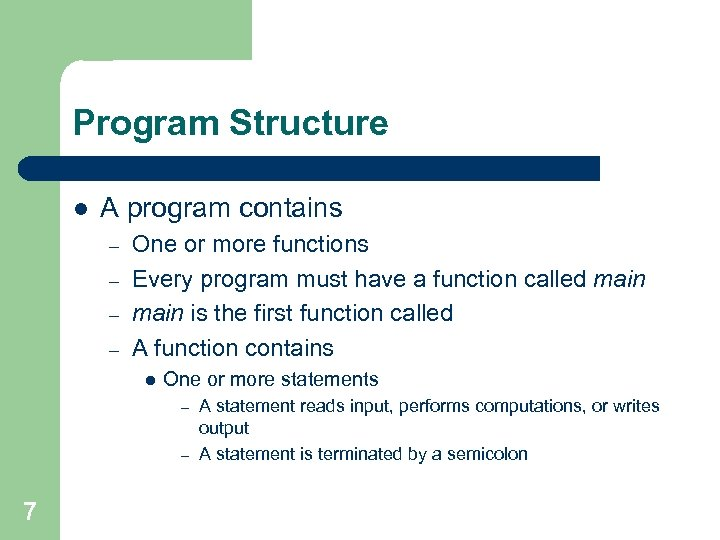Program Structure l A program contains – – One or more functions Every program must have a function called main is the first function called A function contains l One or more statements A statement reads input, performs computations, or writes output – A statement is terminated by a semicolon – 7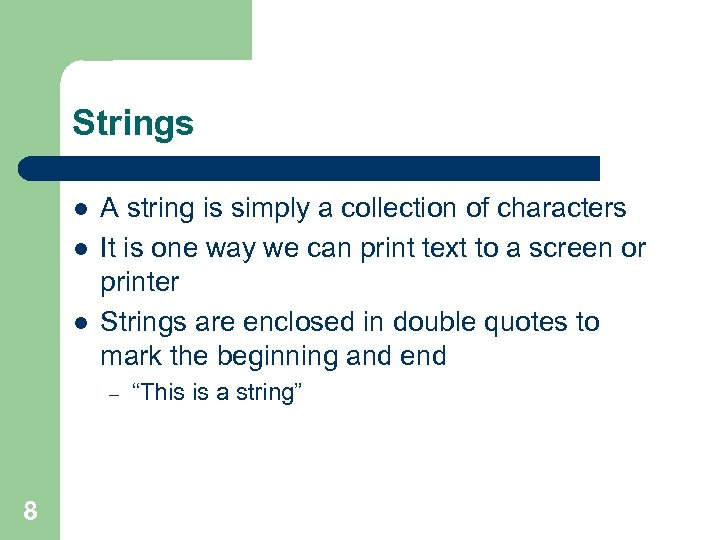Strings l l l A string is simply a collection of characters It is one way we can print text to a screen or printer Strings are enclosed in double quotes to mark the beginning and end – 8 “This is a string”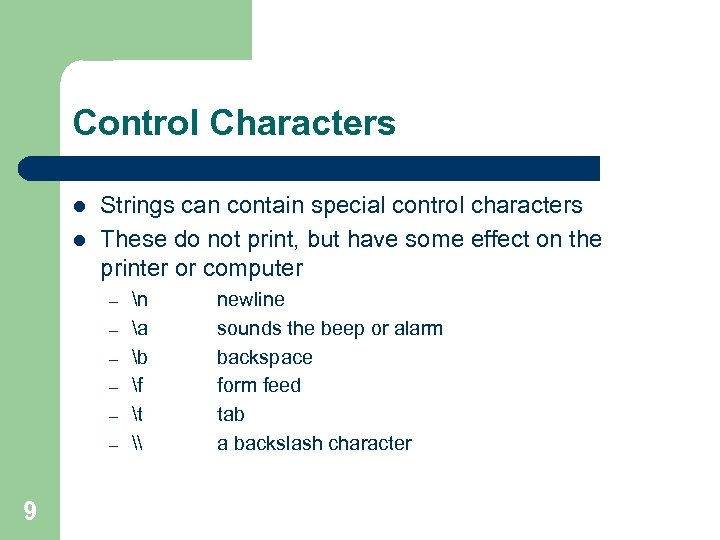Control Characters l l Strings can contain special control characters These do not print, but have some effect on the printer or computer – – – 9 n a b f t \ newline sounds the beep or alarm backspace form feed tab a backslash character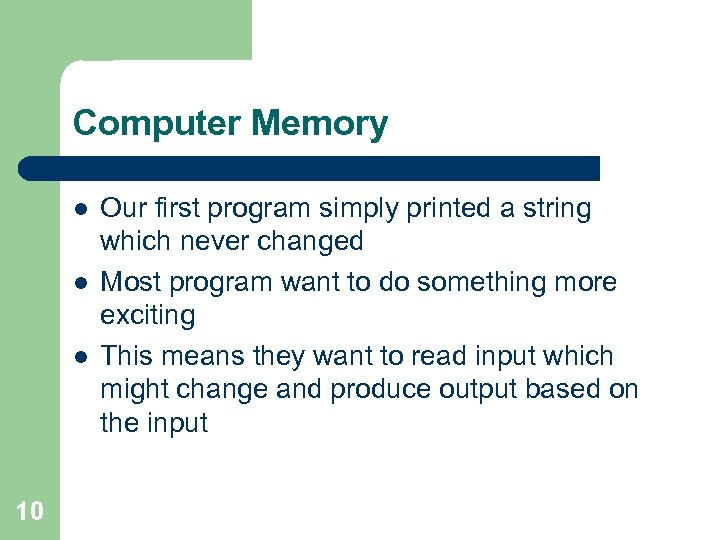Computer Memory l l l 10 Our first program simply printed a string which never changed Most program want to do something more exciting This means they want to read input which might change and produce output based on the input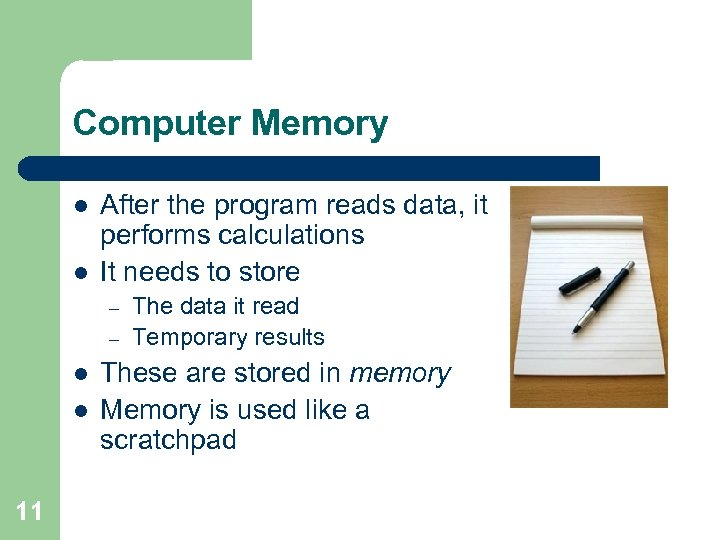Computer Memory l l After the program reads data, it performs calculations It needs to store – – l l 11 The data it read Temporary results These are stored in memory Memory is used like a scratchpad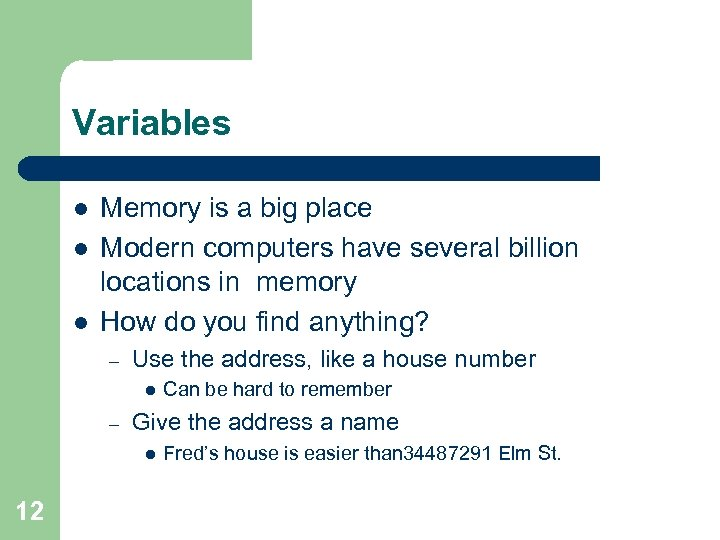Variables l l l Memory is a big place Modern computers have several billion locations in memory How do you find anything? – Use the address, like a house number l – Give the address a name l 12 Can be hard to remember Fred’s house is easier than 34487291 Elm St.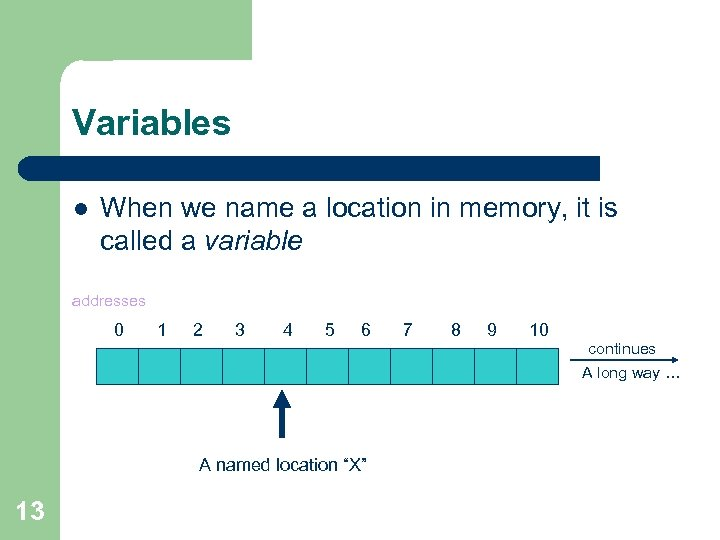Variables l When we name a location in memory, it is called a variable addresses 0 1 2 3 4 5 6 A named location “X” 13 7 8 9 10 continues A long way …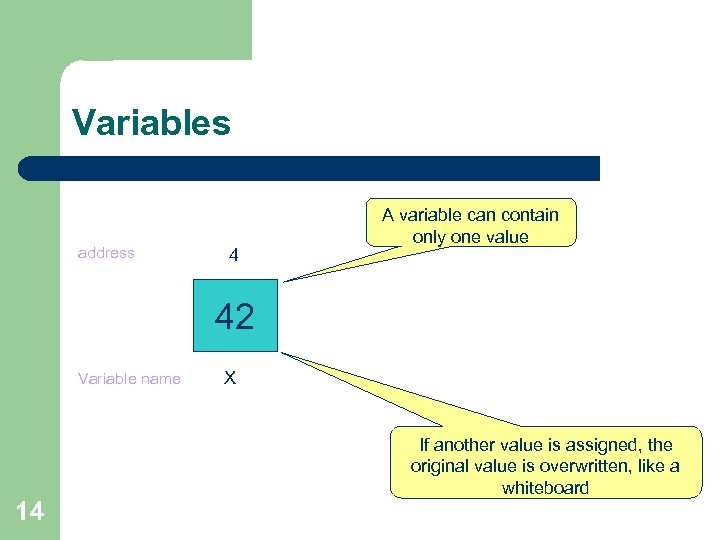Variables address 4 A variable can contain only one value 42 Variable name 14 X If another value is assigned, the original value is overwritten, like a whiteboard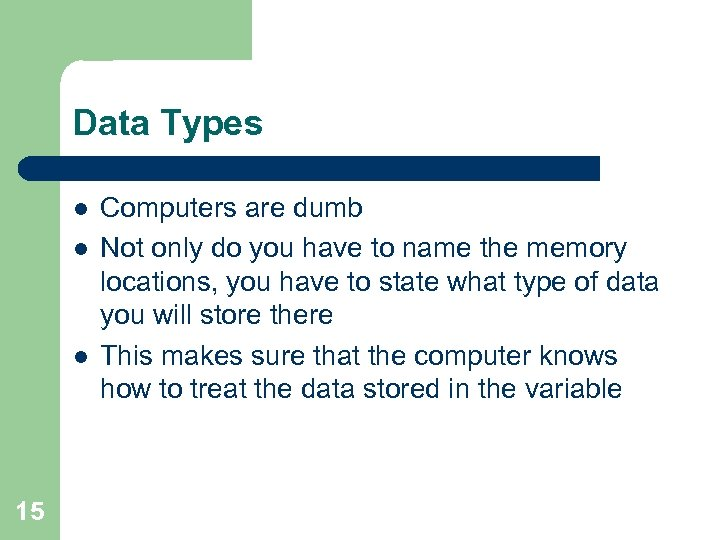Data Types l l l 15 Computers are dumb Not only do you have to name the memory locations, you have to state what type of data you will store there This makes sure that the computer knows how to treat the data stored in the variable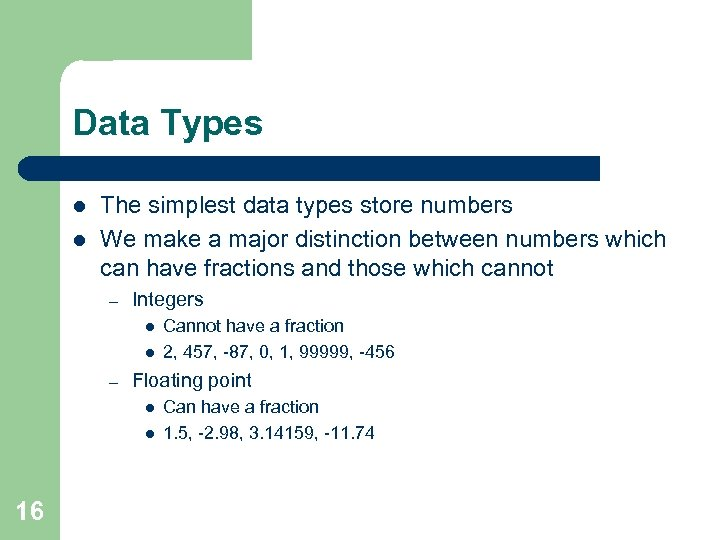Data Types l l The simplest data types store numbers We make a major distinction between numbers which can have fractions and those which cannot – Integers l l – Floating point l l 16 Cannot have a fraction 2, 457, -87, 0, 1, 99999, -456 Can have a fraction 1. 5, -2. 98, 3. 14159, -11. 74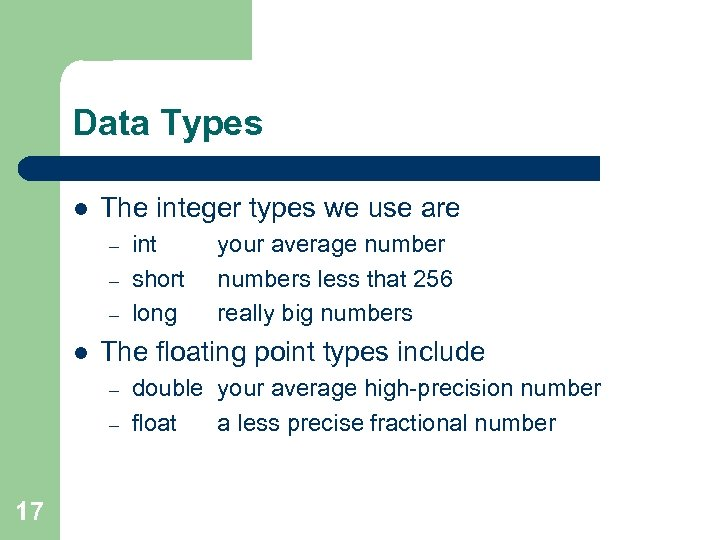Data Types l The integer types we use are – – – l your average numbers less that 256 really big numbers The floating point types include – – 17 int short long double your average high-precision number float a less precise fractional number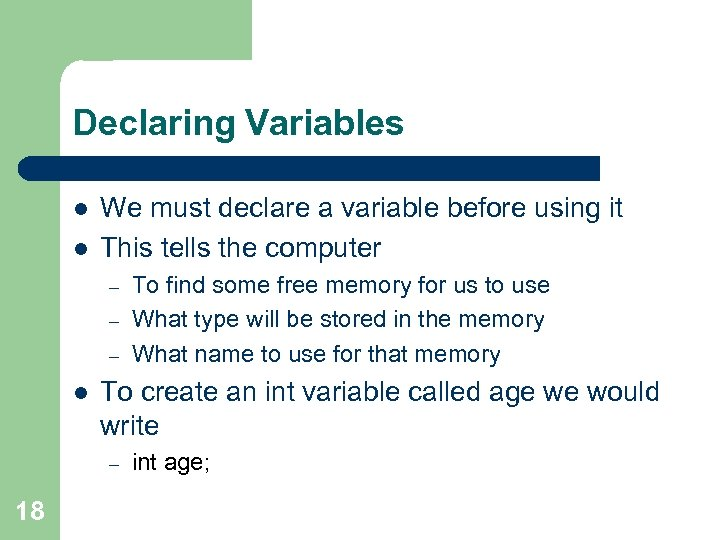Declaring Variables l l We must declare a variable before using it This tells the computer – – – l To create an int variable called age we would write – 18 To find some free memory for us to use What type will be stored in the memory What name to use for that memory int age;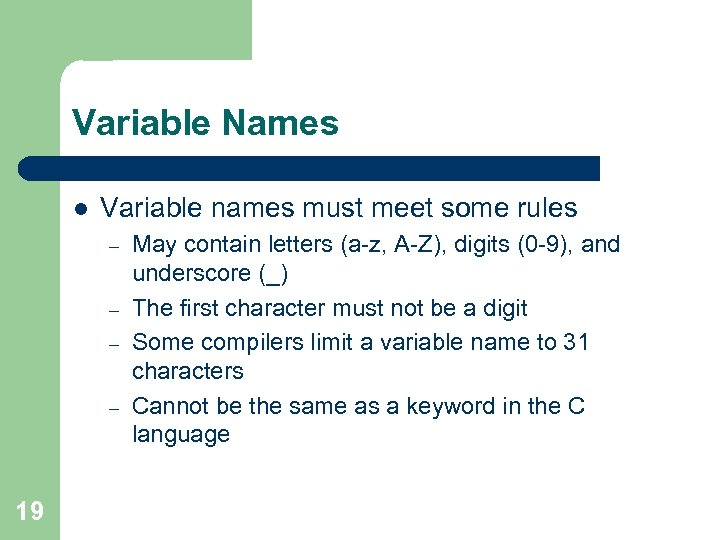Variable Names l Variable names must meet some rules – – 19 May contain letters (a-z, A-Z), digits (0 -9), and underscore (_) The first character must not be a digit Some compilers limit a variable name to 31 characters Cannot be the same as a keyword in the C language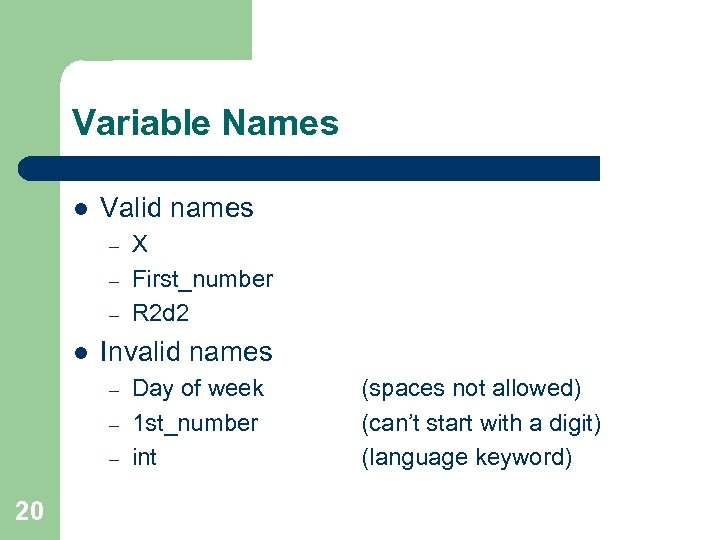Variable Names l Valid names – – – l Invalid names – – – 20 X First_number R 2 d 2 Day of week 1 st_number int (spaces not allowed) (can’t start with a digit) (language keyword)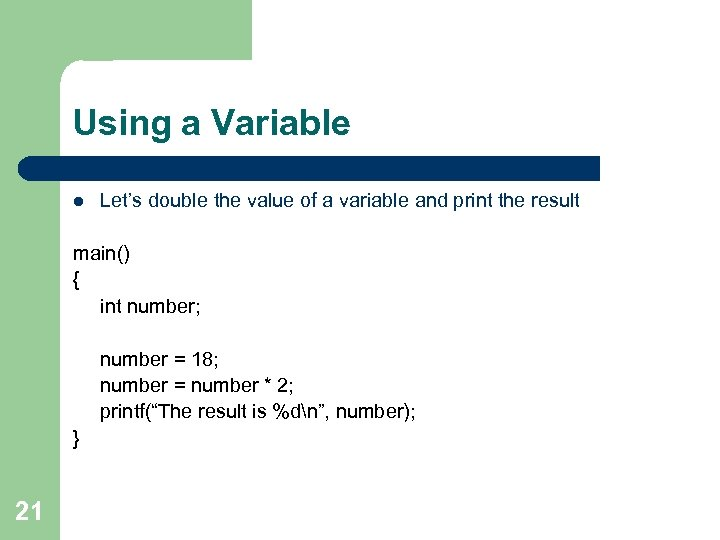Using a Variable l Let’s double the value of a variable and print the result main() { int number; number = 18; number = number * 2; printf(“The result is %dn”, number); } 21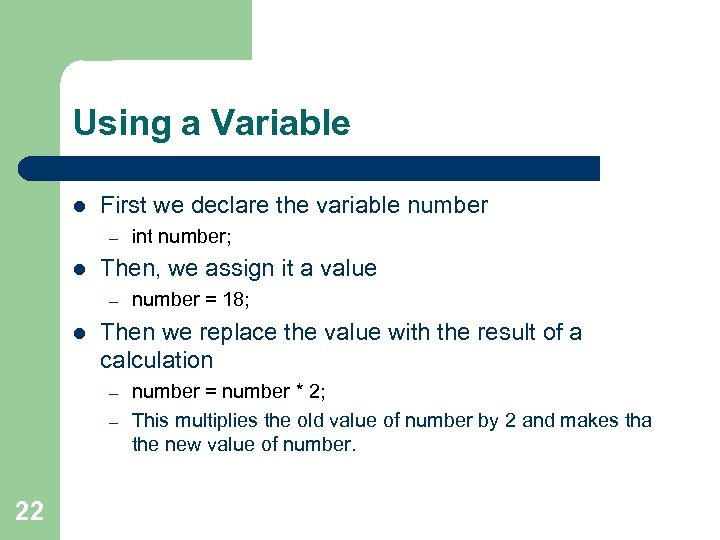Using a Variable l First we declare the variable number – l Then, we assign it a value – l number = 18; Then we replace the value with the result of a calculation – – 22 int number; number = number * 2; This multiplies the old value of number by 2 and makes tha the new value of number.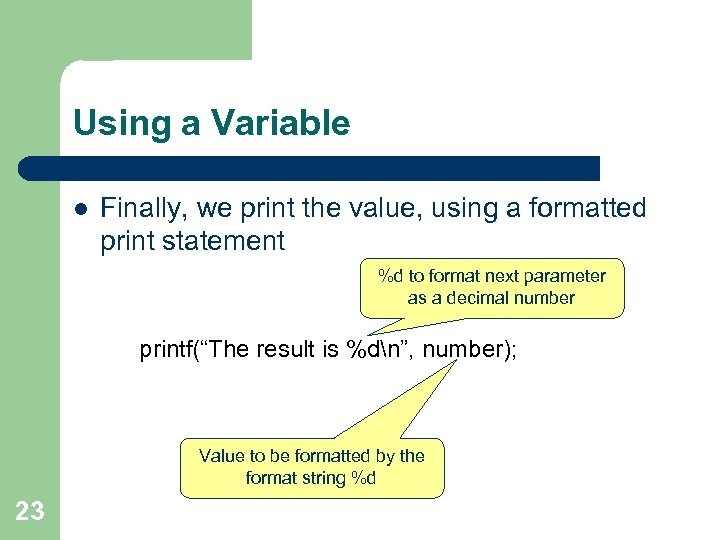Using a Variable l Finally, we print the value, using a formatted print statement %d to format next parameter as a decimal number printf(“The result is %dn”, number); Value to be formatted by the format string %d 23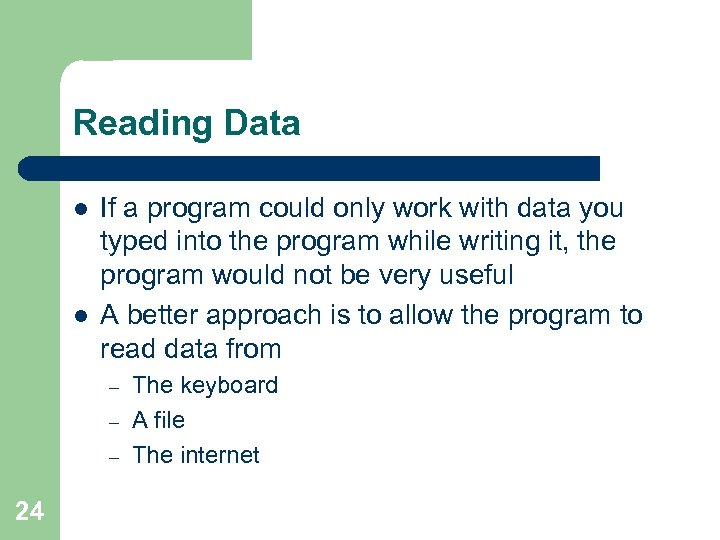Reading Data l l If a program could only work with data you typed into the program while writing it, the program would not be very useful A better approach is to allow the program to read data from – – – 24 The keyboard A file The internet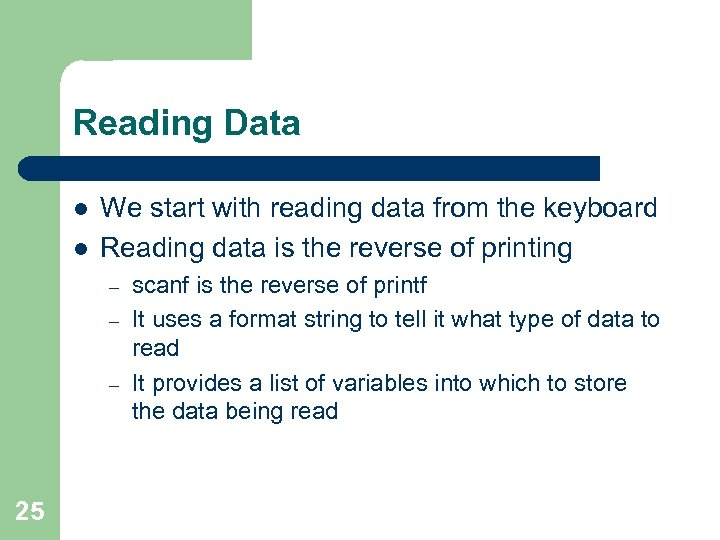Reading Data l l We start with reading data from the keyboard Reading data is the reverse of printing – – – 25 scanf is the reverse of printf It uses a format string to tell it what type of data to read It provides a list of variables into which to store the data being read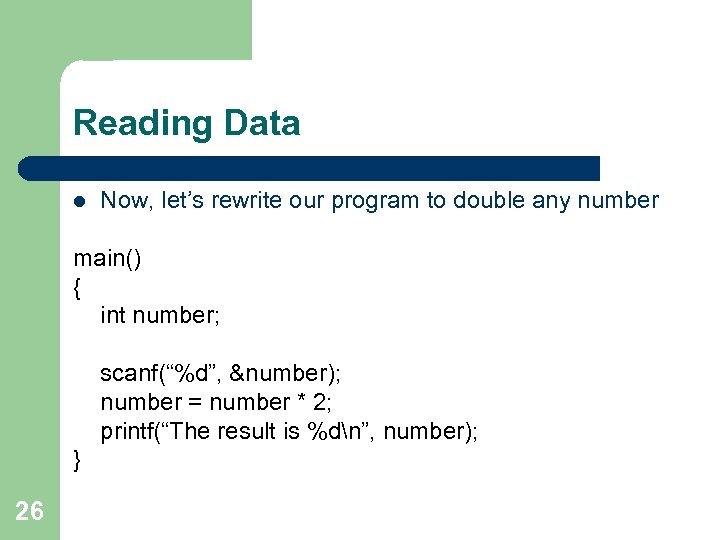Reading Data l Now, let’s rewrite our program to double any number main() { int number; scanf(“%d”, &number); number = number * 2; printf(“The result is %dn”, number); } 26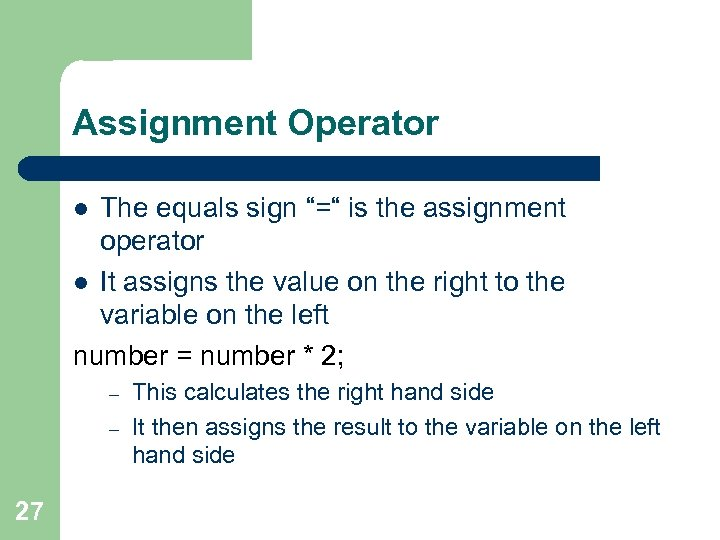Assignment Operator The equals sign “=“ is the assignment operator l It assigns the value on the right to the variable on the left number = number * 2; l – – 27 This calculates the right hand side It then assigns the result to the variable on the left hand side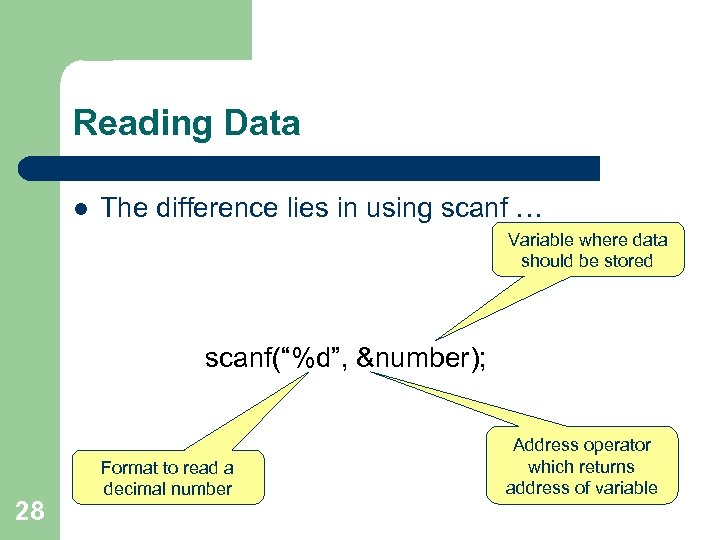Reading Data l The difference lies in using scanf … Variable where data should be stored scanf(“%d”, &number); 28 Format to read a decimal number Address operator which returns address of variable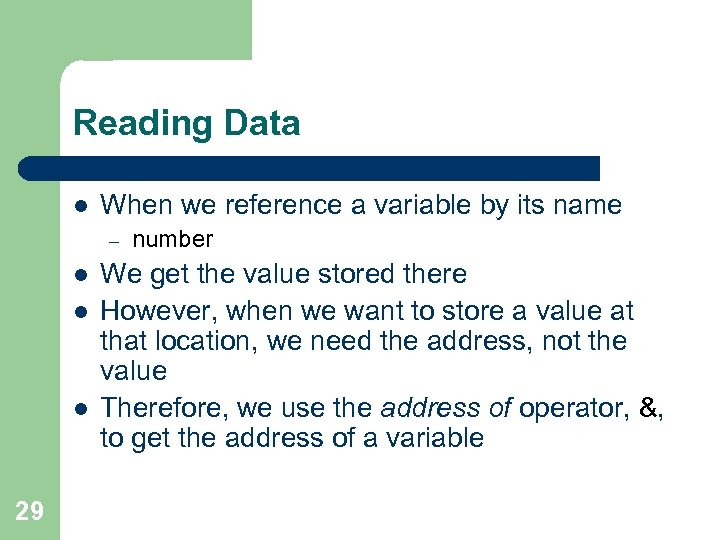Reading Data l When we reference a variable by its name – l l l 29 number We get the value stored there However, when we want to store a value at that location, we need the address, not the value Therefore, we use the address of operator, &, to get the address of a variable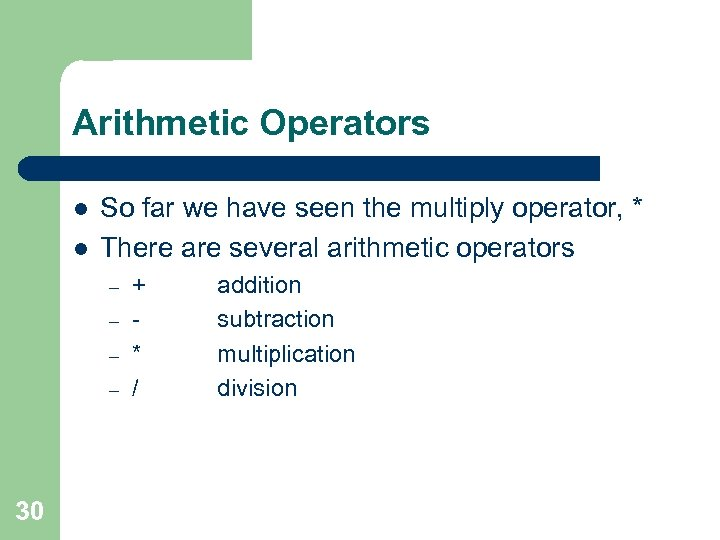Arithmetic Operators l l So far we have seen the multiply operator, * There are several arithmetic operators – – 30 + * / addition subtraction multiplication division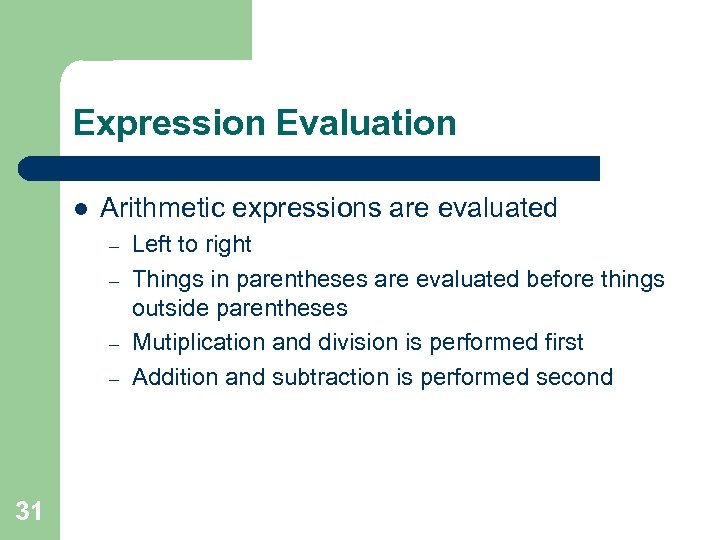Expression Evaluation l Arithmetic expressions are evaluated – – 31 Left to right Things in parentheses are evaluated before things outside parentheses Mutiplication and division is performed first Addition and subtraction is performed second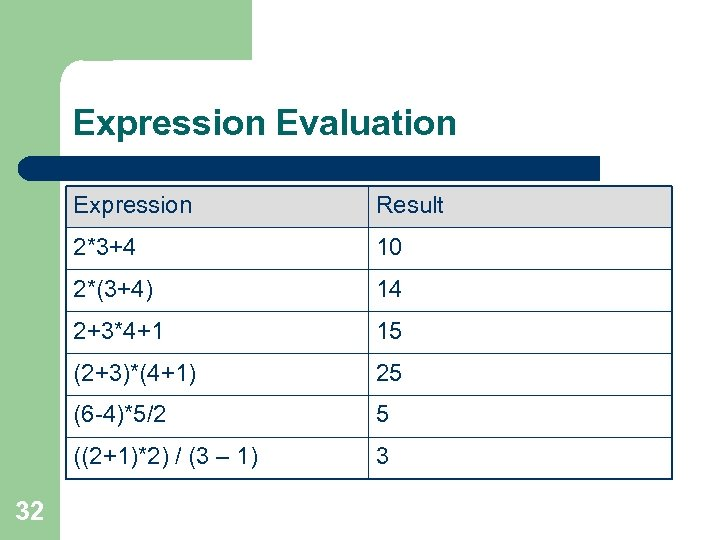Expression Evaluation Expression 2*3+4 10 2*(3+4) 14 2+3*4+1 15 (2+3)*(4+1) 25 (6 -4)*5/2 5 ((2+1)*2) / (3 – 1) 32 Result 3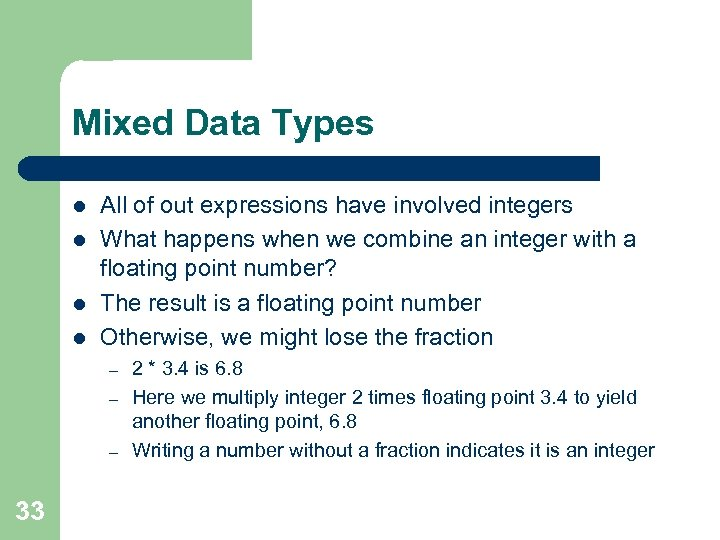Mixed Data Types l l All of out expressions have involved integers What happens when we combine an integer with a floating point number? The result is a floating point number Otherwise, we might lose the fraction – – – 33 2 * 3. 4 is 6. 8 Here we multiply integer 2 times floating point 3. 4 to yield another floating point, 6. 8 Writing a number without a fraction indicates it is an integer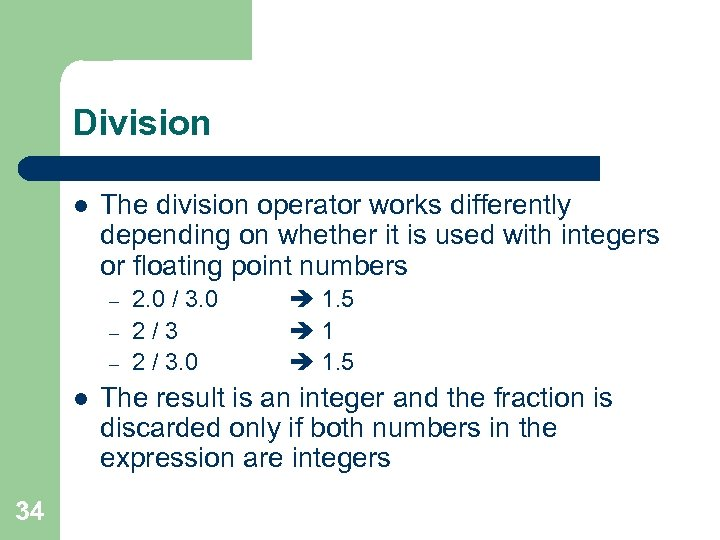Division l The division operator works differently depending on whether it is used with integers or floating point numbers – – – l 34 2. 0 / 3. 0 2/3 2 / 3. 0 1. 5 1 1. 5 The result is an integer and the fraction is discarded only if both numbers in the expression are integers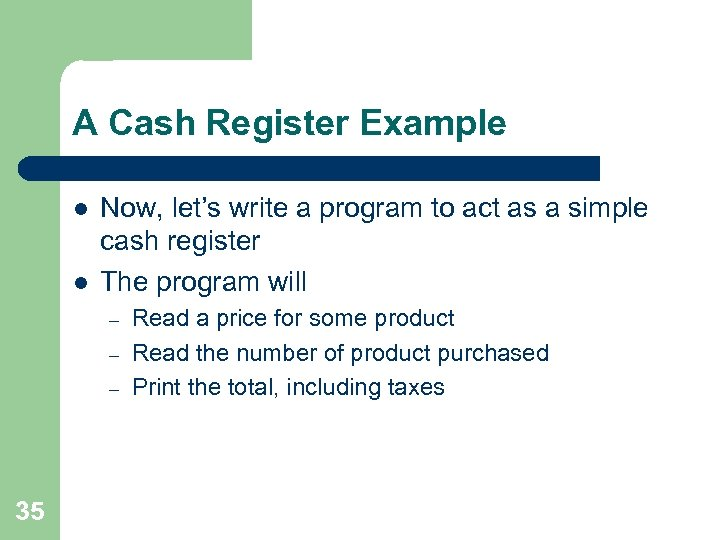A Cash Register Example l l Now, let’s write a program to act as a simple cash register The program will – – – 35 Read a price for some product Read the number of product purchased Print the total, including taxes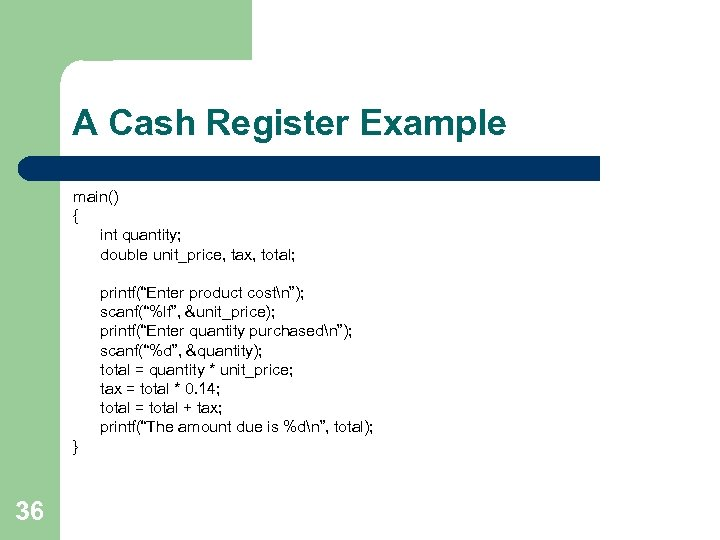A Cash Register Example main() { int quantity; double unit_price, tax, total; printf(“Enter product costn”); scanf(“%lf”, &unit_price); printf(“Enter quantity purchasedn”); scanf(“%d”, &quantity); total = quantity * unit_price; tax = total * 0. 14; total = total + tax; printf(“The amount due is %dn”, total); } 36